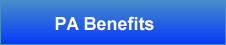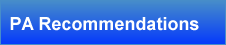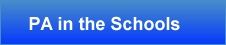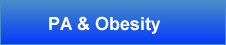Buttons Navigation by Vista-Buttons.com v5.7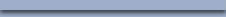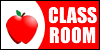Card Play

Suggested Grade Level(s): 1, 2

Content Standard(s):

• 1 - Math
• Use place value understanding and prop. of operations to +/- | 1.NBT.4:
Add within 100, including adding a two-digit number and a one-digit number, and adding a two-digit number and a multiple of 10, using concrete models or drawings and strategies based on place value, properties of operations, and/or the relationship between addition and subtraction; relate the strategy to a written method and explain the reasoning used. Understand that in adding two-digit numbers, one adds tens and tens, ones and ones; and sometimes it is necessary to compose a ten.
• 2 - Math
• Use place value understanding and properties of operations to +/- | 2.NBT.6:
Add up to four two-digit numbers using strategies based on place value and properties of operations.

Purpose of Activity (objectives, etc.):

Students will use playing cards to create an addition or subtraction equation.

Materials/Equipment (Needed):

• Deck of Playing Cards

Description:

1. Teacher takes out all the kings, queens, and jacks from playing cards.
That leaves about 40 cards in the deck. Teacher distributes two cards to each student and has the students stand up next to their desks.
2. Teacher gives the go signal and students begin circulating around the room. When the teacher says stop, the teacher calls out a number and the student must find another student to make an equation out of their two cards.
3. For example: The teacher calls out the number 6, and one student has a 2 and a 9 and the other student has a 3 and a 4. The students can use the 2 and the 4, add them together to make 6 (Or they can use the 9-3 to get 6)
4. After making an equation, students start to circulate around the room
again until the teacher says top and calls out another number.

Time:

10 minutes

Assessment Idea:

Check to be sure students are following cues for start and stop.
Check to see that the students are making true equations.

Source: